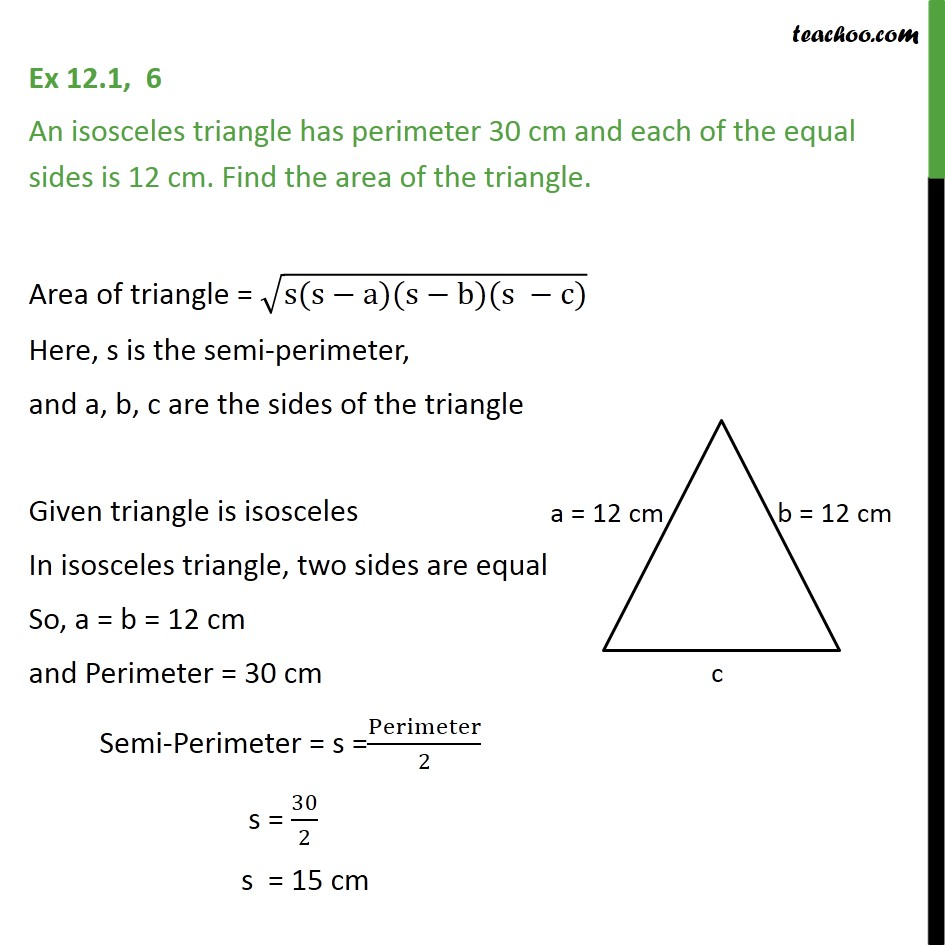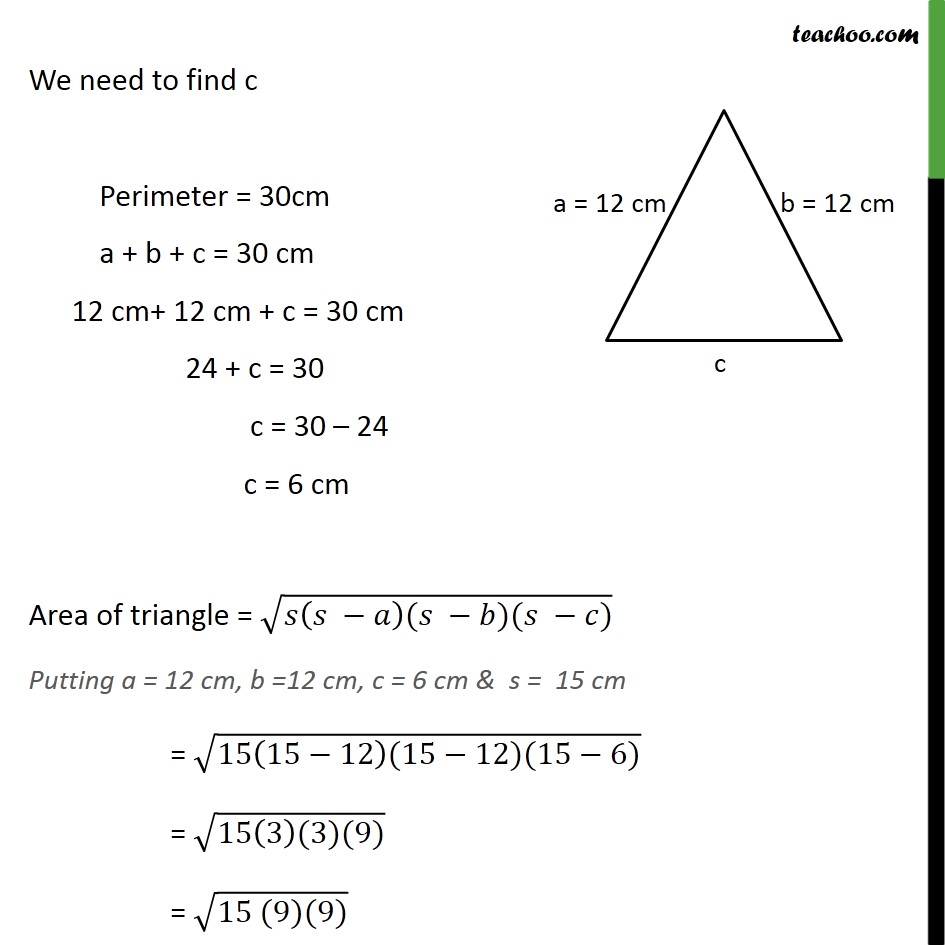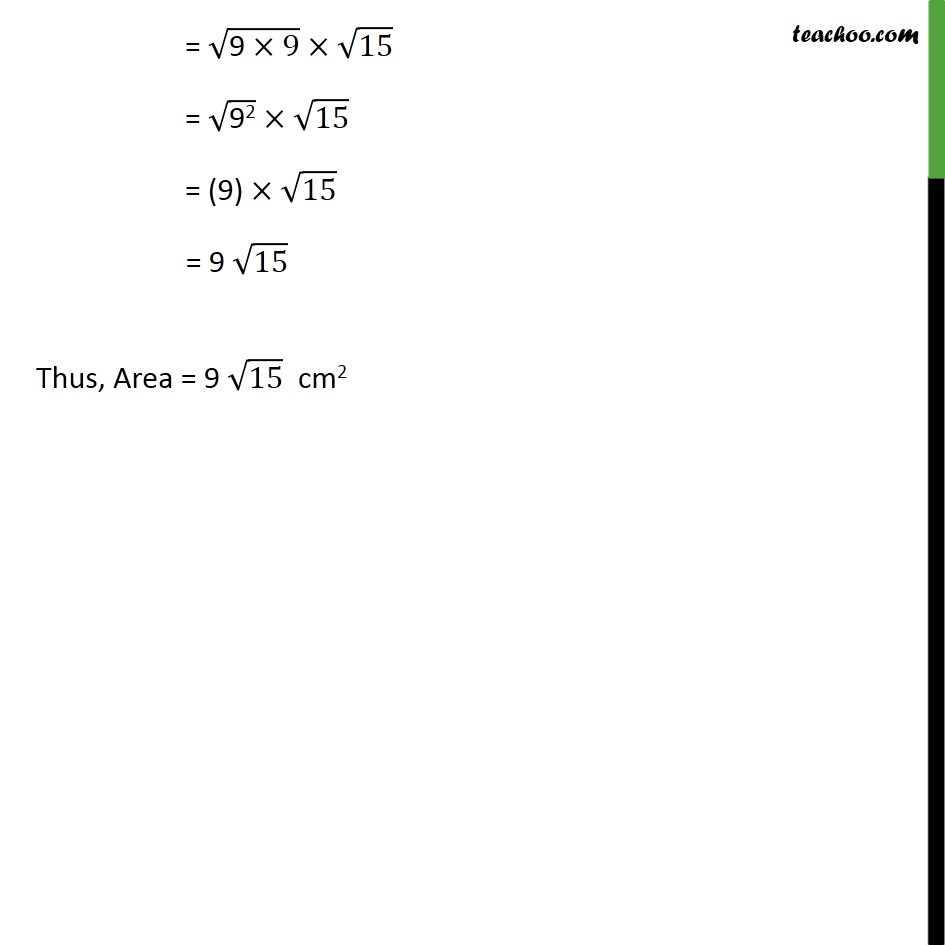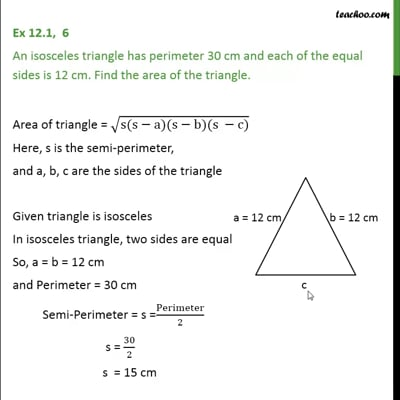Finding area of triangle

Chapter 12 Class 9 Herons Formula
Concept wiseThis video is only available for Teachoo black users

Introducing your new favourite teacher - Teachoo Black, at only ₹83 per month

### Transcript

Ex 12.1, 6 An isosceles triangle has perimeter 30 cm and each of the equal sides is 12 cm. Find the area of the triangle. Area of triangle = (s(s a)(s b)(s c)) Here, s is the semi-perimeter, and a, b, c are the sides of the triangle Given triangle is isosceles In isosceles triangle, two sides are equal So, a = b = 12 cm and Perimeter = 30 cm Semi-Perimeter = s =Perimeter/2 s = 30/2 s = 15 cm We need to find c Perimeter = 30cm a + b + c = 30 cm 12 cm+ 12 cm + c = 30 cm 24 + c = 30 c = 30 24 c = 6 cm Area of triangle = ( ( )( )( )) Putting a = 12 cm, b =12 cm, c = 6 cm & s = 15 cm = (15(15 12)(15 12)(15 6)) = (15(3)(3)(9)) = (15 (9)(9)) = ("9" 9) 15 = ("92" ) 15 = (9) 15 = 9 15 Thus, Area = 9 15 cm2